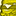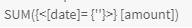# App Development

Announcements
Qlik Cloud Maintenance is scheduled between March 27-30. Visit Qlik Cloud Status page for more details.
cancel
Showing results for
Did you mean:Contributor II

## Sum expression where a date column matches a date variable

I created a variable vLatestDate which is derived from the max month of the [date] columnvLatestDate:

``=Max([date])``

I am now trying to create a column that is a sum expression based on the [date] column matching the vLatestDate variable.

I have tried the follow, but it did not work:

``SUM({<[date]=vLatestDate>} [amount])``

However, the following code does:

``SUM(IF([date]=vLatestDate, [amount])``

Why does the first code not work while the second does?

Labels (1)
• ### General Question

1 Solution

Accepted SolutionsMVP

You seem to have left out the \$() required to parse the variable.

``SUM({<[date]=\$(vLatestDate)>} [amount])``

or

``SUM({<[date]= {'\$(vLatestDate)'} >} [amount])``

4 RepliesMVP

You seem to have left out the \$() required to parse the variable.

``SUM({<[date]=\$(vLatestDate)>} [amount])``

or

``SUM({<[date]= {'\$(vLatestDate)'} >} [amount])``Contributor II
Author

The following code worked:

``SUM({<[date]= {'\$(vLatestDate)'}>} [amount])``Contributor II
Author

Would it be possible to get the [amount] where [date] = vLatestDate - 1 month?

I have tried the following codes but it doesn't work:

``````SUM({<[date]= {'\$(addmonths(vLatestDate, -1))'}>} [amount])
``````

and

``````SUM({<[date]= {\$(addmonths(vLatestDate, -1))}>} [amount])
``````

the expression shows blank values:MVP

Have a look at e.g. this thread for examples of using an expression in the set analysis comparison.

https://community.qlik.com/t5/QlikView-App-Dev/Using-an-expression-within-Set-Analysis/m-p/910261Tags
Community Browser